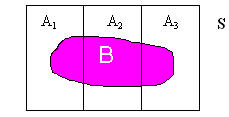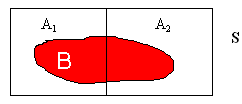LEGACY CONTENT. If you are looking for Voteview.com, PLEASE CLICK HERE

This site is an archived version of Voteview.com archived from University of Georgia on May 23, 2017. This point-in-time capture includes all files publicly linked on Voteview.com at that time. We provide access to this content as a service to ensure that past users of Voteview.com have access to historical files. This content will remain online until at least January 1st, 2018. UCLA provides no warranty or guarantee of access to these files.

### 45-733 PROBABILITY AND STATISTICS I Practice Midterm

Probability and Statistics                      Name__________________________
Spring 1999 Flex-Mode and Flex-Time 45-733
Practice Midterm
Keith Poole

(10 Points)

1. How many ways can 10 people be seated around a circular table, taking into account only the relative location of the 10 people (that is, if all 10 people get up and move one chair to the right, the new arrangement is considered to be the same as the old arrangement)?

9!

Probability and Statistics                      Name__________________________
Spring 1999 Flex-Mode and Flex-Time 45-733
Practice Midterm
Keith Poole

(10 Points)

2. Suppose we have the continuous bivariate probability distribution:
```
æ (3x - y)/5  1 < x < 2
f(x,y) = ç             1 < y < 3
è   0 otherwise
```
1. Find f1(x) and f2(y)

f1(x) = ò-¥+¥ f(x,y)dy = ò13 [(3x - y)/5]dy = (1/5)[3xy - y2/2]|13 = (1/5)[(9x - 9/2) - (3x - 1/2)] = (6x - 4)/5
f2(y) = ò-¥+¥ f(x,y)dx = ò12 [(3x - y)/5]dx = (1/5)[3x2/2 - yx]|12 = (1/5)[(6 - 2y) - (3/2 - y)] = (9/2 - y)/5

Therefore:
```
æ (6x - 4)/5   1 < x < 2
f1(x) = ç
è   0 otherwise

æ (9/2 - y)/5  1 < y < 3
f2(y) = ç
è   0 otherwise
```

2. Are X, Y independent?

NO. Clearly f(x,y) ¹ f1(x)f2(y)

Probability and Statistics                      Name__________________________
Spring 1999 Flex-Mode and Flex-Time 45-733
Practice Midterm
Keith Poole

(10 Points)

3. Suppose 4 people are playing a game with a standard deck of 52 playing cards (as defined in class). If the 52 cards are randomly distributed to the 4 persons and each person receives exactly 13 cards, what is the probability that person A receives 5 spades, person B receives 4 spades, person C receives 3 spades, and person D receives 1 spade?

```
æ   13  öæ   39    ö
ç       ÷ç         ÷
è5 4 3 1øè8 9 10 12ø
P(E) = -----------------
æ     52    ö
ç           ÷
è13 13 13 13ø
```

Probability and Statistics                      Name__________________________
Spring 1999 Flex-Mode and Flex-Time 45-733
Practice Midterm
Keith Poole

(10 Points)

4. Suppose we have a continuous probability distribution
```
æ 2(1 - x)  0 < x < 1
f(x) = ç
è   0 otherwise
```
1. Find F(x).

F(x) = P(X £ x) = ò0x 2(1 - t)dt = (2t - 2t2/2)|0x = 2x - x2

Hence:
```
æ   0      x < 0
ç
F(x) = ç 2x - x2  0 £ x £ 1
ç
è   1      x > 1
```

2. Find P(1/2 < X < 1)

P(1/2 < X < 1) = F(1) - F(1/2) = 1 - 3/4 = 1/4

Probability and Statistics                      Name__________________________
Spring 1999 Flex-Mode and Flex-Time 45-733
Practice Midterm
Keith Poole

(10 Points)

5. Suppose we have the discrete bivariate probability distribution

```
æ c(3y - x)  x=2,3
f(x,y) = ç            y=2,3,4
è   0 otherwise
```
1. Find c

```
y
2   3   4
------------
2 | 4   7  10 | 21
x    |           |
3 | 3   6   9 | 18
|           |
---------------
7  13  19 | 39
```
Therefore, c = 1/39

2. Find E(X) and E(Y).

E(X) = åi=1,n åj=1,m xif(xi, yj) = åi=1,n xif1(xi) = 2*(21/39) + 3*(18/39) = 96/39
E(Y) = åj=1,m åi=1,n yjf(xi, yj) = åj=1,m yjf2(yj) = 2*(7/39) + 3*(13/39) + 4*(19/39) = 129/39

3. Are X, Y Independent.

NO. f(2,2) = 4/39 ¹ f1(2)*f2(2) = (21/39)*(7/39)

Probability and Statistics                      Name__________________________
Spring 1999 Flex-Mode and Flex-Time 45-733
Practice Midterm
Keith Poole

(10 Points)

6. An investor is interested in the stock of Amazon.Com. The investor feels that if the stock market goes up in the next year, the probability is .9 that the price of Amazon.Com stock will go up. If the market goes down, the probability is .4 that Amazon.Com stock will go up. Finally, if the market is unchanged, the probability is .7 that Amazon.Com stock will go up. In addition, the investor thinks that the probabilities are .5, .3, and .2 for the market to go up, go down, or remain unchanged. At the end of the year, the price of Amazon.Com stock has not gone up. What is the probability that the stock market went up?

Let A1 = "Market Goes Up", A2 = "Market Goes Down", A3 = "Market Remains Unchanged",
and let B = "Amazon.Com Stock Does Not go Up".
Graphically, this is:P(A1 | B) = P(A1)P(B | A1)/ [P(A1)P(B | A1) + P(A2)P(B | A2) + P(A3)P(B | A3)] =
(.5*.1)/(.5*.1 + .3*.6 + .2*.3)= .05/.29 = 5/29

Probability and Statistics                      Name__________________________
Spring 1999 Flex-Mode and Flex-Time 45-733
Practice Midterm
Keith Poole

(10 Points)

7. Suppose we own a retail store and we keep track of the number of customer arrivals per hour. Let X be the number of customer arrivals per hour. Over a long period of time we find that:
```
æ .1  x = 0
ç
ç .2  x = 1
ç
ç .3  x = 2
ç
f(x) = ç .2  x = 3
ç
ç .1  x = 4
ç
ç .1  x = 5
ç
è  0 otherwise
```
Find E(X) and VAR(X).

E(X) = åi=1,n xif(xi) = 0*.1 + 1*.2 + 2*.3 + 3*.2 + 4*.1 + 5*.1 = 2.3

E(X2) = åi=1,n xi2f(xi) = 02*.1 + 12*.2 + 22*.3 + 32*.2 + 42*.1 + 52*.1 = 7.3

Hence, VAR(X) = 7.3 - 2.32 = 2.01

Probability and Statistics                      Name__________________________
Spring 1999 Flex-Mode and Flex-Time 45-733
Practice Midterm
Keith Poole

(15 Points)

8. Suppose we have an electric circuit with 6 identical relays. The probability that a relay works when it is activated is .9 and the failures of the relays are independent. Let X be the number of open paths between A and B when the relays are activated. Find the probability distribution of X.
```
|--1--2--3--|
|           |
A--|-----4-----|--B
|           |
|--5-----6--|
```

Clearly, X = 0, 1, 2, 3
P(X = 0) = P[(top path fails) Ç (middle path fails) Ç (bottom path fails)]
P(top path fails) = P(at least one fails)
= 1 - .93 = .271
P(middle path fails) = .1
P(bottom path fails) = 1 -.92 = .19
Hence, P(Y = 0) = .271*.1*.19 = .005149

P(X = 1) = .93*.1*(1 - .92) + (1 - .93)*.9*(1 - .92) + (1 - .93)*.1*.92 = .082143

P(X = 2) = .93*.9*(1 - .92) + .93*.1*.92 + (1 - .93)*.9*.92 = .381267

P(X = 3) = .93*.9*.92 = .96 = .531441
```
æ  .005149  x = 0
ç
ç  .082143  x = 1
ç
f(x) = ç  .381267  x = 2
ç
ç  .531441  x = 3
ç
è    0 otherwise
```

Probability and Statistics                      Name__________________________
Spring 1999 Flex-Mode and Flex-Time 45-733
Practice Midterm
Keith Poole

(10 Points)

9. We toss a coin 4 times. What is the probability we get 4 heads given that we get at least 2 heads?

Let X be the number of heads. Therefore

P(X = 4 | X ³ 2) = P(X = 4 Ç X ³ 2) /P(X ³ 2) = P(X = 4)/P(X ³ 2) = P(X = 4)/[1 - P(X = 0) - P(X = 1)] =
[(1/2)4]/[1 - (1/2)4 - (4 choose 1)(1/2)1(1/2)3] = (1/16)/[1 - 1/16 - 4/16] = 1/11

Probability and Statistics                      Name__________________________
Spring 1999 Flex-Mode and Flex-Time 45-733
Practice Midterm
Keith Poole

(10 Points)

10. Suppose that the student population at Carnegie-Mellon is 65% male and 35% female and that 30% of the males smoke and that 20% of the females smoke. We randomly draw a student from this population and learn that the person smokes. What is the probability that the person is male?

Let A1 be "male", A2 be "female", and B be "smoker". Graphically this is:P(A1) = .65, P(A2) = .35, P(B | A1) = .3, P(B | A2) = .2

P(A1 | B) = P(A1)P(B | A1)/ [P(A1)P(B | A1) + P(A2)P(B | A2)] =
(.65*.3)/(.65*.3 + .35*.2) = .195/(.195 + .07) » .736